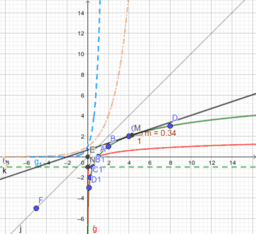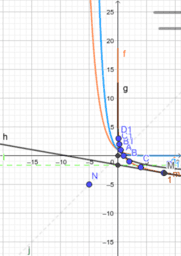# Lesson Plan: Logarithmic function. Properties.

This book contains the lesson project that introduces the logarithmic function and discovers the properties of this function.
•### Logarithmic function. Properties.

•### The geometric representation of the graph of the logarithmic base function supraunitary

•### The geometric representation of the graph of the logarithmic base function subunitary

•